# NCERT SOLUTIONS FOR CLASS 8 MATHS CHAPTER 14 FACTORISATION

The National Council of Educational Research and Training (NCERT) is an autonomous body of the Indian government that formulates the curricula for schools in India that are governed by the Central Board of Secondary Education (CBSE) and certain state boards. Therefore, students who will be taking the Class 10 tests administered by various boards should consult this NCERT Syllabus in order to prepare for those examinations, which in turn will assist those students get a passing score.

You can also download the NCERT SOLUTIONS FOR CLASS 8 MATHS CHAPTER 14 FACTORISATION to assist you in comprehensively reviewing the course material and achieving a higher score on your upcoming assessments.

Students are able to create a strong conceptual basis with the help of the NCERT Solutions PDFs available at SWC. This base plays a key part in the students’ preparation for the later phases of the competitive examinations. We give comprehensive answers to challenges posed by NCERT in accordance with the methodology provided by CBSE. The students are able to effortlessly prepare all of the ideas that are presented in their respective courses better and more successfully, and they are even able to succeed in passing the most difficult competitive examinations, such as JEE Main, JEE Advanced, NEET, AIMS, and so on.

Your academic journey begins a new stage as you reach the eighth grade, and a new facet of learning awaits you. This is the year where pupils begin to acquire an interest in the topic at hand, and it is also the year in which you choose whether you will study business, humanity, or science in the following year (class 12). As a consequence of this, having a solid foundation of knowledge in fields such as mathematics and physics comes highly recommended. The NCERT textbook for class 8 is the greatest book now available on the market for enhancing one’s education in the areas of mathematics and science. Read up on the theory that is presented in the book for grade 8 and answer all of the questions that are posed in the practice section of the NCERT textbook.

When working through the exercises in the NCERT textbook, if you run into any type of difficulty or uncertainty, you may use the swc NCERT Solutions for class 8 as a point of reference. While you are reading the theory from textbook, it is imperative that you always have notes prepared. You should make an effort to understand things from the very beginning so that you may create a solid foundation in the topic. Use the NCERT as your parent book to ensure that you have a strong foundation. After you have finished reading the theoretical section of the textbook, you should go to additional reference books.

## NCERT SOLUTIONS FOR CLASS 8 MATHS CHAPTER 14 FACTORISATION – Exercises

### NCERT Solutions for Class 8 Maths Exercise 14.1

Question 1.

Find the common factors of the given terms.

Solution :

(i) 12x = 2 × 2 × 3

36 = 2 × 2 × 3 × 3

Hence, the common factors are 2, 2 and 3 = 2 × 2 × 3 = 12

(ii)   2y = 2 ×y

22xy = 2 × 11 × x × y

Hence the common factors are

2 and y  =  2 × y = 2y

(iii)   14 pq = 2×7× p × q

Question 2.

Factorize the following expressions.

Solution :

Taking common factors from each term,

Question 3.

Factorize:

Solution :

NCERT Solutions for Class 8 Maths Exercise 14.2

Question 1.

Factorize the following expressions:

Solution :

Question 2.

Factorize

(i)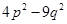(ii)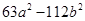(iii)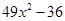(iv)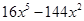(v)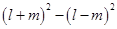(vi)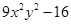(vii)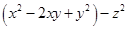(viii)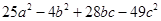Solution :

Question 3.

Factorize the expressions:

Solution :

Question 4.

Factorize:

Solution :

(iv)

Question 5.

Factorize the following expressions:

Solution :

NCERT Solutions for Class 8 Maths Exercise 14.3

Question 1.

Carry out the following divisions:

Solution :

Question 2.

Divide the given polynomial by the given monomial:

Solution :

Question 3.

Work out the following divisions:

Solution :

(iii)

Solution :

Solution :

Question 13.

Solution :

Solution :

L.H.S. =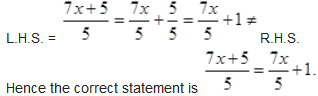R.H.S.

## Conclusions for NCERT Solutions for Class 8 Maths Chapter 14

SWC academic staff has developed NCERT answers for the 14th chapter of the eighth-grade mathematics curriculum. We have solutions prepared for all of the exercises in the 14th chapter. The answers, broken down into steps, to all of the questions included in the NCERT textbook’s first chapter are provided here. Read the 14th chapter on theory. Be certain that you have read the theory section of chapter14 of the NCERT textbook and that you have learned the formulas for the chapter that you are studying.